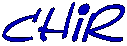# Function-Plot Applet

Function-Plot is a small Java Applet that draws the curve of any given function.

Directly type any function, like SIN(1/x) and press ENTER.

The following classic functions are recognised by the expression parser :

sin(x)
cos(x)
tan(x)
asin(x)
acos(x)
atan(x)
exp(x)
log(x)
Neperian logarithm
abs(x)
Absolute value
sqrt(x)
Square root

The following operators are recognised :